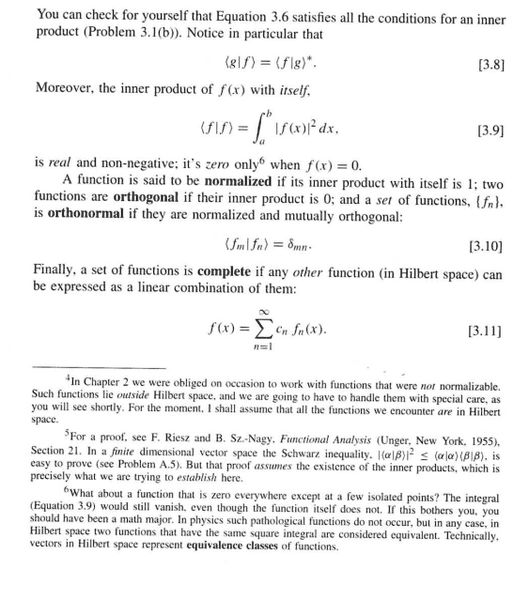# Equivalent vectors in a Hilbert space

bob900
In Griffith's intro to QM it says on page 95 (in footnote 6) :

"In Hilbert space two functions that have the same square integral are considered equivalent. Technically, vectors in Hilbert space represent equivalence classes of functions."

But that means that if we take for example

f(x) = 1 | 0 < x < 1

and

g(x) = $\sqrt{1/10}$ | 0 < x < 10

both f(x) and g(x) have the same square integral ∫|f(x)|^2 dx = ∫|g(x)|^2 dx = 1

But how can they be considered "equivalent" - if they are wavefunctions for a particle, for example, then they represent completely different probability predictions! f(x) says you can find a particle with equal probability in [0,1], while for g(x) it's [0,10]

Jazzdude
Either Griffith is wrong or you must have misunderstood something, but that statement is clearly untrue.

Vectors in the hilbert space describe the same state if they are related by multiplication of a nonzero complex number. That means physical states are really rays in the hilbert space. Mathematically this is the projective space H/~ where ~ is the equivalence relation that identifies identical states.

Homework Helper
It's a very unfortunate statement, to say the least. It should be a transcription of Jazzdude's 2nd paragraph in post #2 in terms of a specific Hilbert space (L^2(over what??)), but it's wrongly worded.

Scratch that and get a better book.

P.S. There's no footnote on page 95 of my version of Griffiths (1995, 1st edition I guess).

bob900
P.S. There's no footnote on page 95 of my version of Griffiths (1995, 1st edition I guess).

Here it is :bob900
So...A vector space has to have a null vector. If L2(-inf,+inf) functions form the vector space, the only null vector is the f(x) = 0 function.

But also for any vector space, <f|f> has to be >0 for any non null vector. And yet, here
for a function like

g(x) = 1 | x=1
g(x) = 0 | everywhere else

(and other such functions)

<g|g>=0 even though clearly g is not a null vector.

So either we exclude functions like g from the vector space, or we define the vector space to be the set of equivalence classes of functions (where all functions like g would be the same class). But what is the precise formulation/definition of such an equivalence relation?

So either we exclude functions like g from the vector space, or we define the
vector space to be the set of equivalence classes of functions (where all
functions like g would be the same class). But what is the precise
formulation/definition of such an equivalence relation?
The functions are "equivalent" if they differ only on a set of Lebesgue measure 0.
(Isolated points in the domain of a function are of measure 0.)

http://en.wikipedia.org/wiki/Lebesgue_integral
http://en.wikipedia.org/wiki/Lebesgue_measure

P.S., The wording of Griffith's footnote
Griffiths said:
In Hilbert space two functions that have the same square integral are considered equivalent.
is wrong. But,
Griffiths said:
Technically, vectors in Hilbert space represent equivalence classes of functions.
is ok.